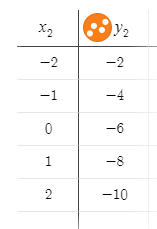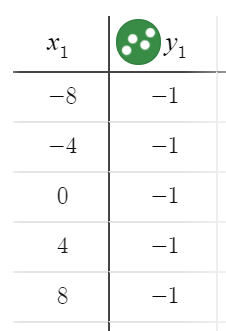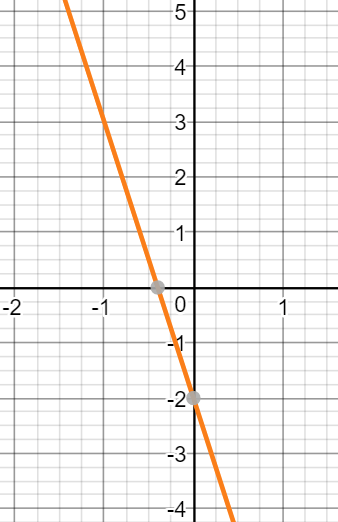# LINR 1 | Lesson 3 | Practice (Increasing or Decreasing Solutions)

 1.Decreasing The $$y$$-values decrease as the $$x$$-values increase. 2.Increasing  The $$y$$-values increase as the $$x$$-values increase. 3.  $$y=\dfrac{1}{3}x-4$$ Increasing The slope is positive. 4.Decreasing Order the $$x$$-values from least to greatest to show that the $$y$$-values decrease. 5.Undefined The $$y$$-values vary as the $$x$$-values remain constant. 6.  $$y=-3x+2$$ Decreasing The slope is negative. 7.Constant The $$y$$-values are constant as the $$x$$-values increase. 8.Decreasing The $$y$$-values decrease as the $$x$$-values increase.